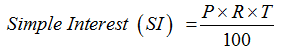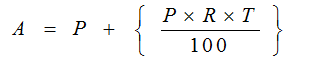Friday , March 22 2019
Home / Simple Interest | Basic Concepts# Simple Interest | Basic Concepts

The idea of simple interest and compound interest is particularly relevant to the universe of banking and financial aspects. At whatever point we obtain a certain amout of money, we pay back the first sum went with a specific measure of interest on that sum. As it were, those are the charges of getting that amount of money. Simple interest is one method of determining the amount due at the end of loan duration. Another method of interest application is compound interest, but we study about it in next article.

Simple Interest Tooltip1: The Definitions
Principal (P): The original sum of money loaned/deposited. Also known as capital.
Interest (I): The amount of money that you pay to borrow money or the amount of money that you earn on a deposit.
Time (T): The duration for which the money is borrowed/deposited.
Rate of Interest (R): The percent of interest that you pay for money borrowed, or earn for money deposited

Simple Interest Tooltip 2: The FormulaWhere:
P: Principal (original amount)
R: Rate of Interest (in %)
T: Time period (yearly, half-yearly etc.)

Amount Due at the end of the time period, A = P (original amount) + SIIf you have a close look, Simple Interest is nothing else but an application of the concept of percentages.

Simple Interest Tooltip 3: Basic Problems to explain the concept

Basic Problem 1: What is the SI on Rs. 7500/- at the rate of 12% per annum for 8 years?

Using the Basic Formula:
Simple Interest (SI) = (P x R x T)/100
P – Principal amount, T- Number of years, R – Rate of Interest

Given P = 7500, T = 8 Years, R = 12%
Simple Interest (S.I.) = (7500X12X8)/100
Simple Interest (S.I.) = 7200

Basic Problem 2: A man borrowed Rs 15000/- at the rate of 24% SI and to clear the debt after 6years, much he has to return:

Using the Basic Formula:
Simple Interest (SI) = (P x R x T)/100
P – Principal amount, T- Number of years, R – Rate of Interest
Given P = 15000, T = 6 Years, R = 24%
Simple Interest (S.I.) = (15000X24X6)/100= Rs 21600
Therefore, total interest = 21600
Total repayment = S.I + Principal amount = 21600 + 15000 = Rs 36600

Basic Problem 3: A man borrowed Rs.12000 at the rate of 10% SI, and lent the same sum toanother person at the rate of 15% what will be the gain after 5 years?

Using the Basic Formula:
Simple Interest (S.I.) = (P x R x T)/100
P – Principal amount, T- Number of years, R – Rate of Interest

The man borrowed at 10% and he lent the same sum to another person at 15%
Therefore, his gain is actually equal to the different in the interest rate (per year)
= 15 – 10 =5% for 1 year
Thus, to calculate his gain, we use this difference as the rate of interest.
Given T = 5 years and P = Rs. 12000
Amount Gained = (12000x5x5)/100 = Rs 3000
Therefore, his gain = Rs 3000/-

error: Content is protected !!